### 6.2. Basic guidelines regarding the position of rhematizers in tectogrammatical trees

Nodes representing rhematizers are assigned the functor `RHEM` (see Section 7.5, "RHEM").

The guidelines for positioning of rhematizers in tectogrammatical trees are simple:

• a rhematizer (i.e. the node representing the rhematizer) is placed as the closest left sister (in the underlying word order) of the first node of the expression that is in its scope.

• if the scope of a rhematizer includes the governing predicate, the rhematizer is placed as the closest left daughter of the node representing the governing predicate.

• if a rhematizer constitutes the focus proper, it is placed according to the guidelines for the position of the focus proper (see Section 3.1.1, "Focus proper") - i.e. on the rightmost path leading from the effective root of the tectogrammatical tree.

Scope of a rhematizer. Thus, the position of a rhematizer in the tectogrammatical tree is determined according to over which part of the sentence it has scope. A rhematizer signaling the focus has in principle in its scope all contextually non-bound modifications (including their further modifications) standing to the right from it in the surface structure of the sentence. A rhematizer signaling a contrastive topic has in principle in its scope the first contrastive contextually bound modification (including its further modifications) standing to the right from it. There are, however, complex constructions, mainly within nominal groups (see Section 6.4.2, "Rhematizers in nominal groups"), where the scope of a rhematizer over further modifications (contextually non-bound or contrastive contextually bound) is questionable. It seems that the "strength" of a scope diminishes downwards in the structure of the sentence. These phenomena, however, have not been studied thoroughly.

In the surface structure of the sentence, a rhematizer can be followed by:

• contextually non-bound expressions (nodes representing such expressions are assigned the `tfa` value `f`; see Section 2.2.3, "Contextually non-bound expression (value `f` in attribute `tfa`)").

The rhematizer signals that the contextually non-bound expressions belong to the focus. The scope of the rhematizers usually covers the whole part of the sentence after the rhematizer.

• a contrastive contextually bound expression (a node representing such an expression is assigned the `tfa` value `c`; see Section 2.2.2, "Contrastive contextually bound expression (value `c` in attribute `tfa`)").

The rhematizer signals that the contrastive contextually non-bound expression belongs to the contrastive topic. The scope of the rhematizer usually includes just this expression, possibly its modifications (i.e. its subtree).

• non-contrastive contextually bound expressions (nodes representing such expressions are assigned the `tfa` value `t`; see Section 2.2.1, "Non-contrastive contextually bound expression (value `t` in the attribute `tfa`)").

When the rhematizer is followed by non-contrastive contextually bound expressions, the following cases arise:

• if the rhematizer is followed only by non-contrastive contextually bound expressions and then contextually non-bound expressions, it is probably the case of a non-contact position of the rhematizer: the rhematizer signals the focus (contrastive topic) and its scope begins at the first contextually non-bound (contrastive contextually bound) expression appearing to the right from it.

This case arises mainly in nominal groups. For more information see Section 6.4.2, "Rhematizers in nominal groups".

• if the rhematizer is followed by non-contrastive contextually bound expressions, it could be the case of a so-called second-instance sentence. In such cases is loses its rhematizing function, it is no more relevant for the topic-focus articulation of the sentence. Usually a whole construction comprising a rhematizer is repeated from the preceding text and the rematizer retains the scope from its first occurrence.

• no expression.

When the rhematizer is not followed by any expression in the surface structure of the sentence (the rhematizer is the last expression of the sentence), it is a special case where the rhematizer constitutes the focus proper.

For the scope of syntactic negation with the function of a rhematizer see Section 13.1, "Negating and affirmative expressions as rhematizers".

Compare:

• Jenom.`RHEM` JIRKA to viděl. (=lit. Only George it.ACC saw.)

= Neviděl to nikdo jiný než Jirka. (=No one else than George saw it.)

The scope of the rhematizer is constituted by the Actor Jirka (=George). The node representing the rhematizer is placed as the closest left sister of the Actor Jirka . Cf. Fig. 10.37.

• Odmítl jsem jenom.`RHEM` sedět. (=lit. (I) refused _ only to_sit.)

= Hodlal jsem dělat cokoli jiného, než sedět. (=I intended to do anything else than sitting.)

The scope of the rhematizer is constituted by the Patient sedět (=to_sit). The node representing the rhematizer is placed as the closest left sister of the Patient sedět (=to_sit) . Cf. Fig. 10.38.

• Udělali by to pouze.`RHEM` , kdybych se stavbou souhlasil. (=lit. (They) would do it.Acc only if with (the) construction (I) agreed.)

= Neudělali by to v žádném jiném případě, než kdybych souhlasil. (=They would not do it in any other case except if I agreed.)

The scope of the rhematizer is constituted by the dependent clause kdybych se stavbou souhlasil (=lit. if with (the) construction (I) agreed). The node representing the rhematizer is placed as the closest left sister (in the underlying word order) of the node representing the expression that is in its scope, i.e. as the closest left sister of the node for the verb souhlasit (=to_agree). Cf. Fig. 10.39.

• Udělali by to, kdybych souhlasil pouze.`RHEM` se stavbou. (=lit. (They) would do it.Acc if (I) agreed only with (the) construction.)

= Udělali by to, kdybych nesouhlasil s ničím jiným než se stavbou. (=They would do it if I did not agree with anything else than the construction.)

The scope of the rhematizer is constituted by the Patient of the verb souhlasit (=to_agree). The node representing the rhematizer is placed as the closest left sister of the Patient node se stavbou (=lit. with (the) construction). Cf. Fig. 10.40.

• Chtěl bych jedině.`RHEM` auto na dálkové ovládání. (=lit. (I) would like only (a) car with remote control.)

= Nechci nic jiného než auto na dálkové ovládání. (=I do not want anything else than a car with remote control.)

The scope of the rhematizer comprises the Patient of the verb auto na dálkové ovládání (=lit. (a) car with remote control) . The node representing the rhematizer is placed as the closest left sister of the node representing the expression that is in its scope, i.e. as the closest left sister of the node for the noun auto (=car) . Cf. Fig. 10.41.

• Chtěl bych auto jedině.`RHEM` na dálkové ovládání. (=lit. (I) would like (a) car only with remote control.)

= Nechci jiné auto než na dálkové ovládání. (=I do not want another car than a car with remote control.)

The scope of the rhematizer is constituted only by the modification na dálkové ovládání (=lit. with remote control). The node representing the rhematizer is placed as the closest left sister of the first node representing the expression that is in its scope, i.e. as the closest left sister of the node for the noun ovládání (=control). Cf. Fig. 10.42.

• Karel výklad přímo.`RHEM` hltal. (=lit. Charles (the) exposition.Acc just devoured.)

The scope of the rhematizer is constituted by the governing predicate hltat (to_devour). The node representing the rhematizer is placed as the closest left daughter of the node representing the governing predicate. Cf. Fig. 10.43.

• Karel především.`RHEM` chtěl, abych odešel. (=lit. Charles primarily wanted _ (me) to_leave)

The scope of the rhematizer is comprises both the governing predicate and the whole subordinate clause chtěl, abych odešel (=lit. (he) wanted _ (me) to_leave.) The node representing the rhematizer is placed as the closest left daughter of the node representing the governing predicate chtít (=to_want). Cf. Fig. 10.44.

Figure 10.37. Position of rhematizers in tectogrammatical trees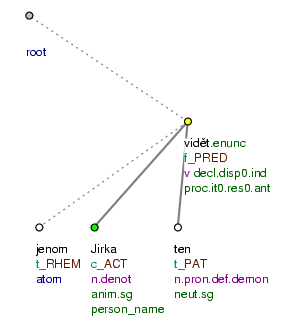Jenom Jirka to viděl. (=lit. Only George it.ACC saw.)

Figure 10.38. Position of rhematizers in tectogrammatical trees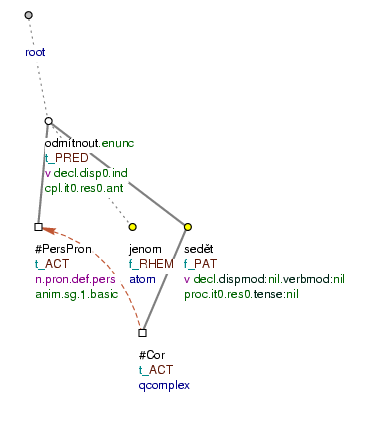Odmítl jsem jenom sedět. (=lit. (I) refused _ only to_sit.)

Figure 10.39. Position of rhematizers in tectogrammatical trees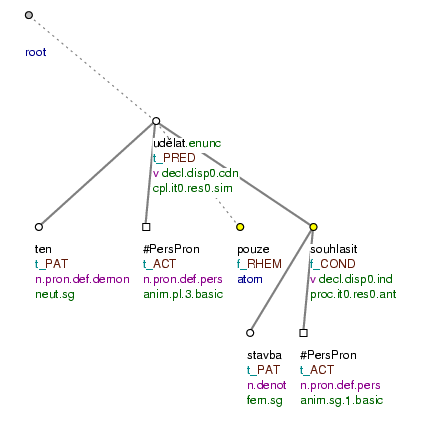Udělali by to pouze, kdybych se stavbou souhlasil. (=lit. (They) would do it.ACC only if with (the) construction (I) agreed.)

Figure 10.40. Position of rhematizers in tectogrammatical trees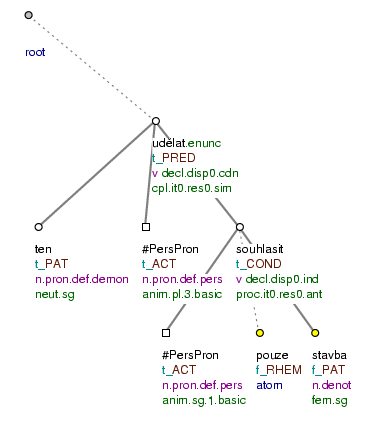Udělali by to, kdybych souhlasil pouze se stavbou. (=lit. (They) would do it.ACC if (I) agreed only with (the) construction.)

Figure 10.41. Position of rhematizers in tectogrammatical trees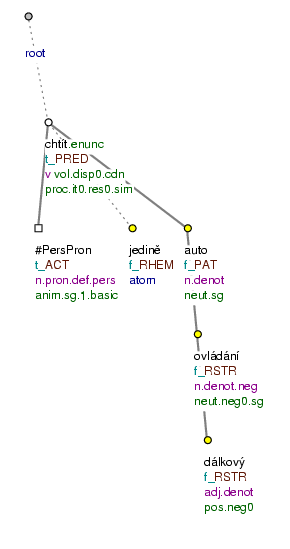Chtěl bych jedině auto na dálkové ovládání. (=lit. (I) would like only (a) car with remote control.)

Figure 10.42. Position of rhematizers in tectogrammatical trees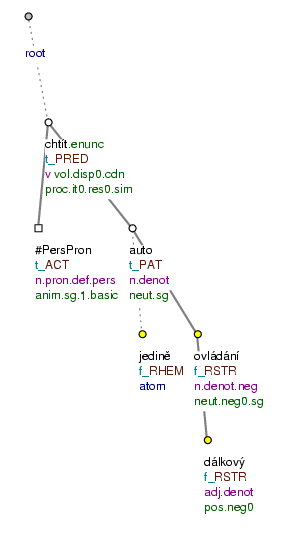Chtěl bych auto jedině na dálkové ovládání. (=lit. (I) would like (a) car only with remote control.)

Figure 10.43. Position of rhematizers in tectogrammatical trees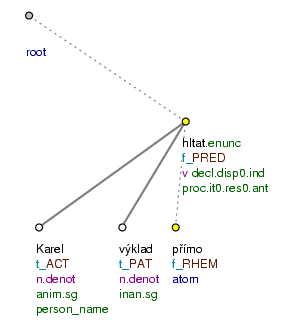Karel výklad přímo hltal. (=lit. Charles (the) exposition.ACC just devoured.)

Figure 10.44. Position of rhematizers in tectogrammatical trees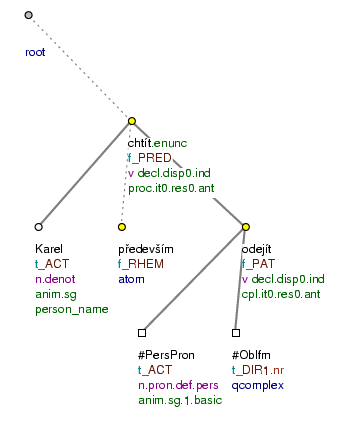Karel především chtěl, abych odešel. (=lit. Charles primarily wanted _ (me) to_leave)